# Chapter 13 - Surface Areas and Volume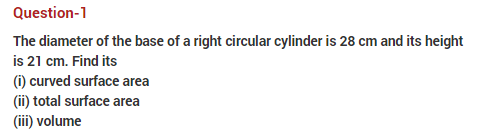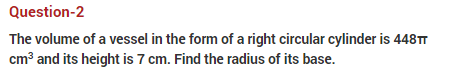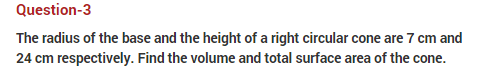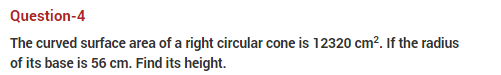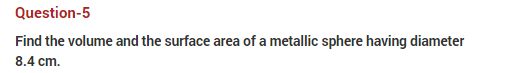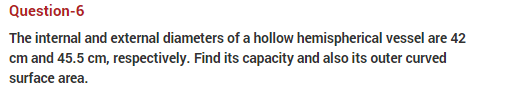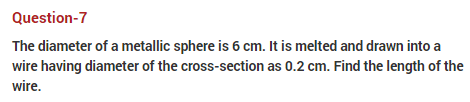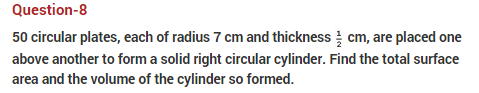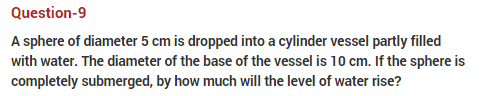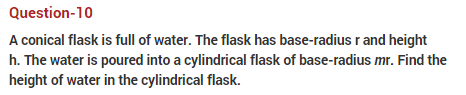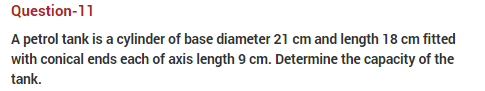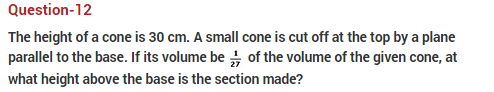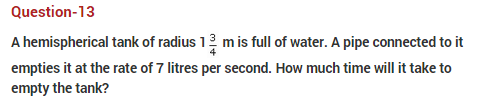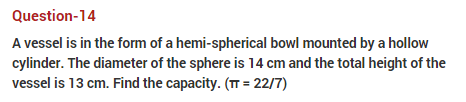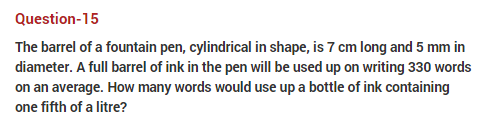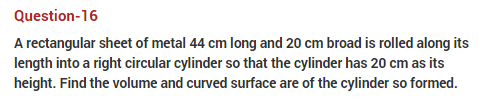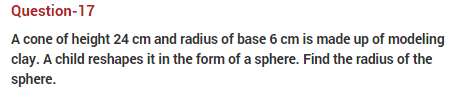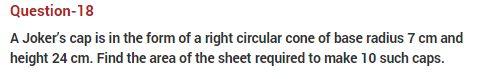#### Practise This Question

Which of the following is not a quadratic equation?
(i) (2x+3)2=3x25x
(ii) 2xx2=x2+5
(iii) (x2+2x)2=x4+3+4x2
(iv) x2+5x = 10